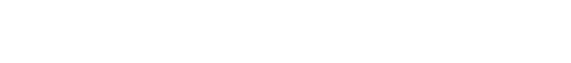# How to Use Today Function in Excel

In this lesson, you will learn how to use the TODAY function. This function is really easy and is more powerful than you thought.

The function, which returns the current system date, is mainly used in various forms. As well as in logistics, e.g., to calculate how many days were left until delivery or transportation day.

## Today function syntax

Syntax is just =TODAY()

Excel will display the current date, e.g., 2013-07-30

As you see above, the TODAY function has no arguments. Its correct result depends on correctly setting the date (and time) in Windows.## How do I calculate how many years have passed?

• Just use this formula: =YEAR(TODAY())-1986

The answer is 27 years. Yes, I am 27 years old.

• =INT((TODAY()-A1)/365.25) - write your date to the A1 cell. It could date from the future as well.
• YEAR(TODAY())-YEAR(A1) - another way to calculate the year difference

## How do I calculate the time difference using the TODAY function?

• =DATEVALUE("2/11/2020")-TODAY() - difference between your date and the current date Remember about the proper formatting of cells? It must be GENERAL or NUMBER.
• =A1<TODAY()-10 - checks if A1 date is earlier than 10 days ago. It could be useful for checking on some payments from clients. Displays TRUE or FALSE.
• =DATEDIF(A1,TODAY(),"y") & " Years, " & DATEDIF(A1,TODAY(),"ym") & " Months" - displays how many years and months passed from A1 date to current date.
• =DATEDIF(A1,TODAY(),"y") & " Years, " & DATEDIF(A1,TODAY(),"ym") & " Months, " & DATEDIF(A1,TODAY(),"md") & " Days" - gives you years, months, and days between two dates.

## Today function examples

### Simple Today Formula

We need to find out today. There are different dates, and we could not find today’s date. We thought we would do ourselves a favor, and just use today's formula.### Today Plus 10 Days

We do have issues figuring out what the date would be ten days from now. This is critical, and we need to find out the today's formula to find the date for that day.### Crazy Date's Calculation

We are trying to find out the date based on different assessments that would allow us to add today to a week ago. This is why we are using today's formula to find the appropriate answer.### Date Value and Today Formula

The situation is that we would like to find out the number of days between today and a certain date in the future. A situation like this makes it important to use both the date values and the today formulas to get the result. It is also critical to format the cell as a number because it would return the total number of days.### DAY and TODAY

We just want to know today's number in the month. It is not about finding out the date, but about today.### Month and Today

We would like to find out the current month of the day. This is why it is important to use both the month and today formulas simultaneously.### Year and Today Formula

The circumstances are quite different when the information we would need is specific. This is because we would like to know the age of the person who is celebrating his birthday today. It would help to write the birthday card.### Double Today Formula

We are trying to find out the process, and we want to know the process time. This is why we are using the double today formula.### Double Today and Month Formula

It is time to find out the period between the today and month formula in the process.### Double Year and Today

We want to find out if we could use the year-to-date formula to find out the number of days within the period.### Other examples of the TODAY function

• =DAY(TODAY()) - extracts current day
• =MONTH(TODAY()) - extracts current month
• =WEEKDAY(TODAY()) - extracts the number of the day of the week
• =TEXT(WEEKDAY(TODAY()),"dddd") - displays name of the day of the week
• =DATE(YEAR(TODAY()),MONTH(TODAY())+1,1)-1 - displays the last day of current month
• =IF(TODAY()=A1,TRUE,FALSE) - checks if A1 is current date and displays TRUE or FALSE

Note that the TODAY function is a volatile function, which means it will recalculate every time the worksheet is opened or recalculated, even if there are no changes to the worksheet.